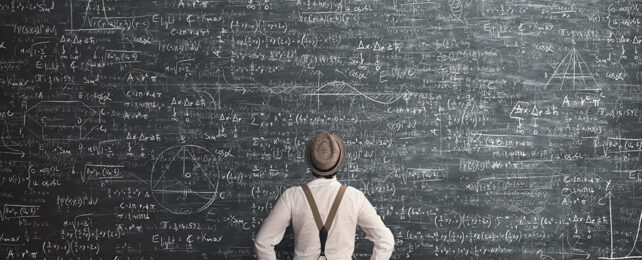## Τρίτη 21 Νοεμβρίου 2023

### Mathematicians Discover The Ninth Dedekind Number, After 32 Years of Searching

Undeterred after three decades of looking, and with some assistance from a supercomputer, mathematicians have finally discovered a new example of a special integer called a Dedekind number.Only the ninth of its kind, or D(9), it is calculated to equal 286 386 577 668 298 411 128 469 151 667 598 498 812 366, if you're updating your own records. This 42 digit monster follows the 23-digit D(8) discovered in 1991.

Grasping the concept of a Dedekind number is difficult for non-mathematicians, let alone working it out. In fact, the calculations involved are so complex and involve such huge numbers, it wasn't certain that D(9) would ever be discovered.

"For 32 years, the calculation of D(9) was an open challenge, and it was questionable whether it would ever be possible to calculate this number at all," says computer scientist Lennart Van Hirtum, from the University of Paderborn in Germany.

At the center of a Dedekind number are Boolean functions, or a kind of logic that selects an output from inputs made up of just two states, such as a true and a false, or a 0 and a 1.

#### Δημοσίευση σχολίου